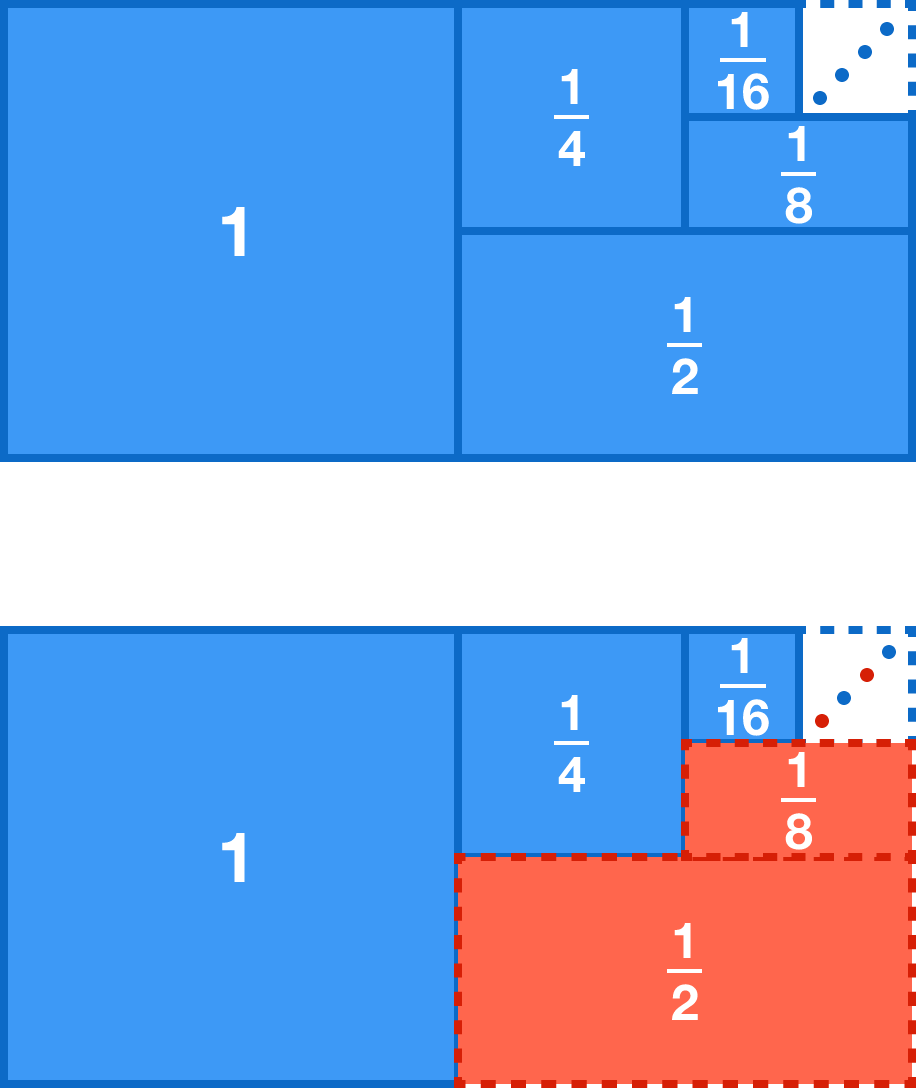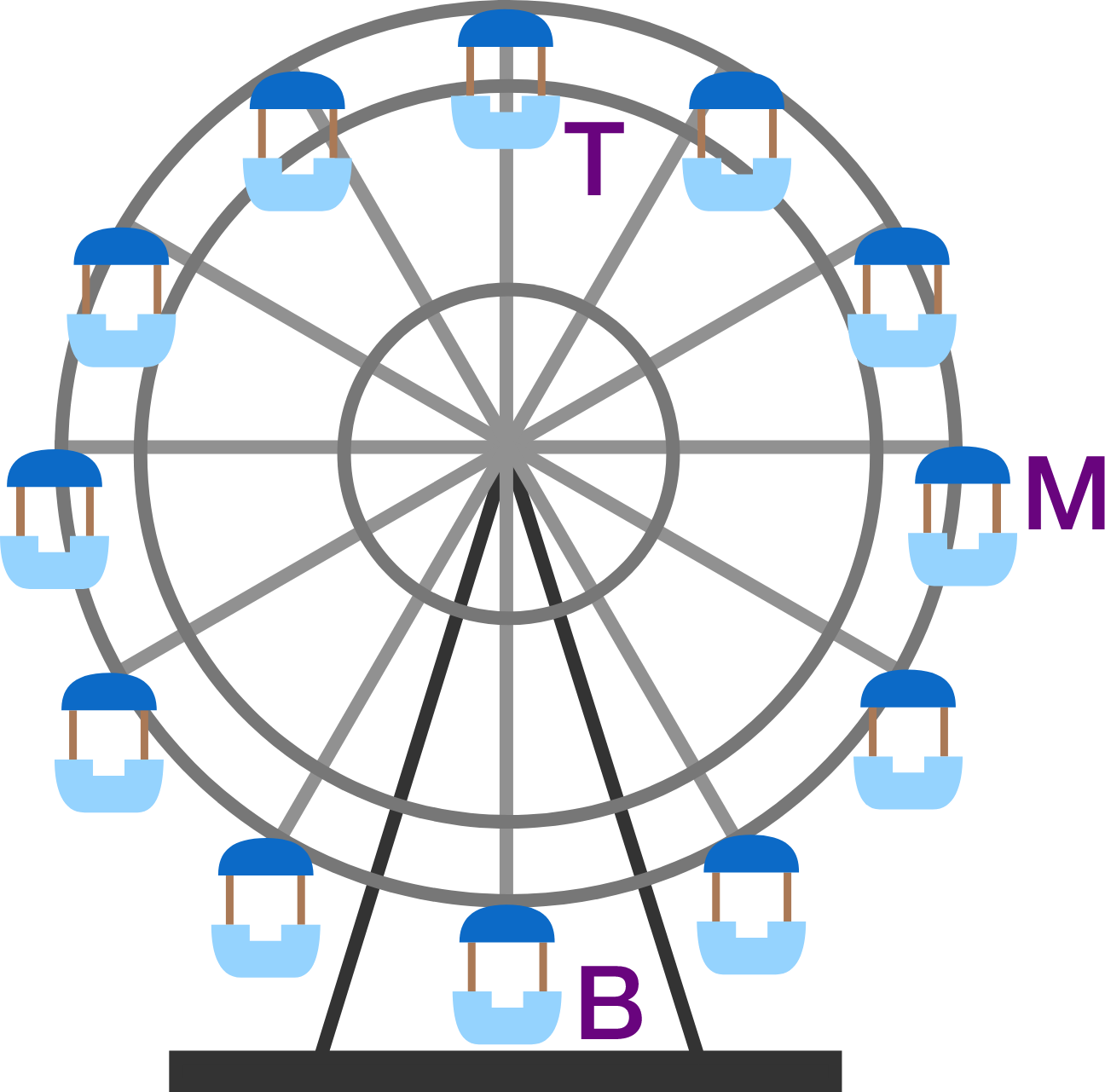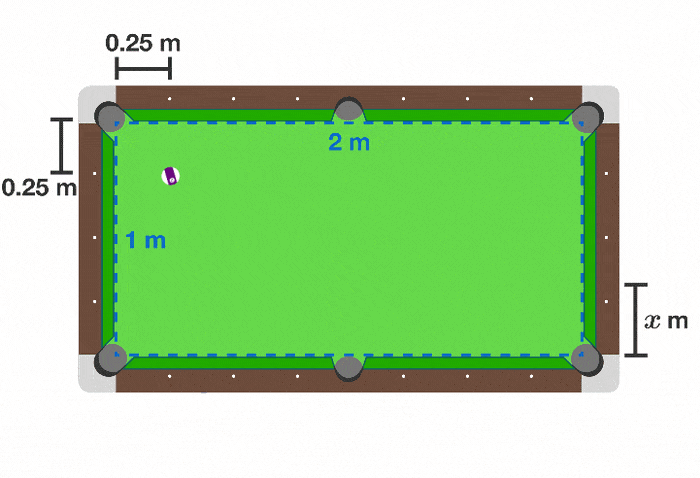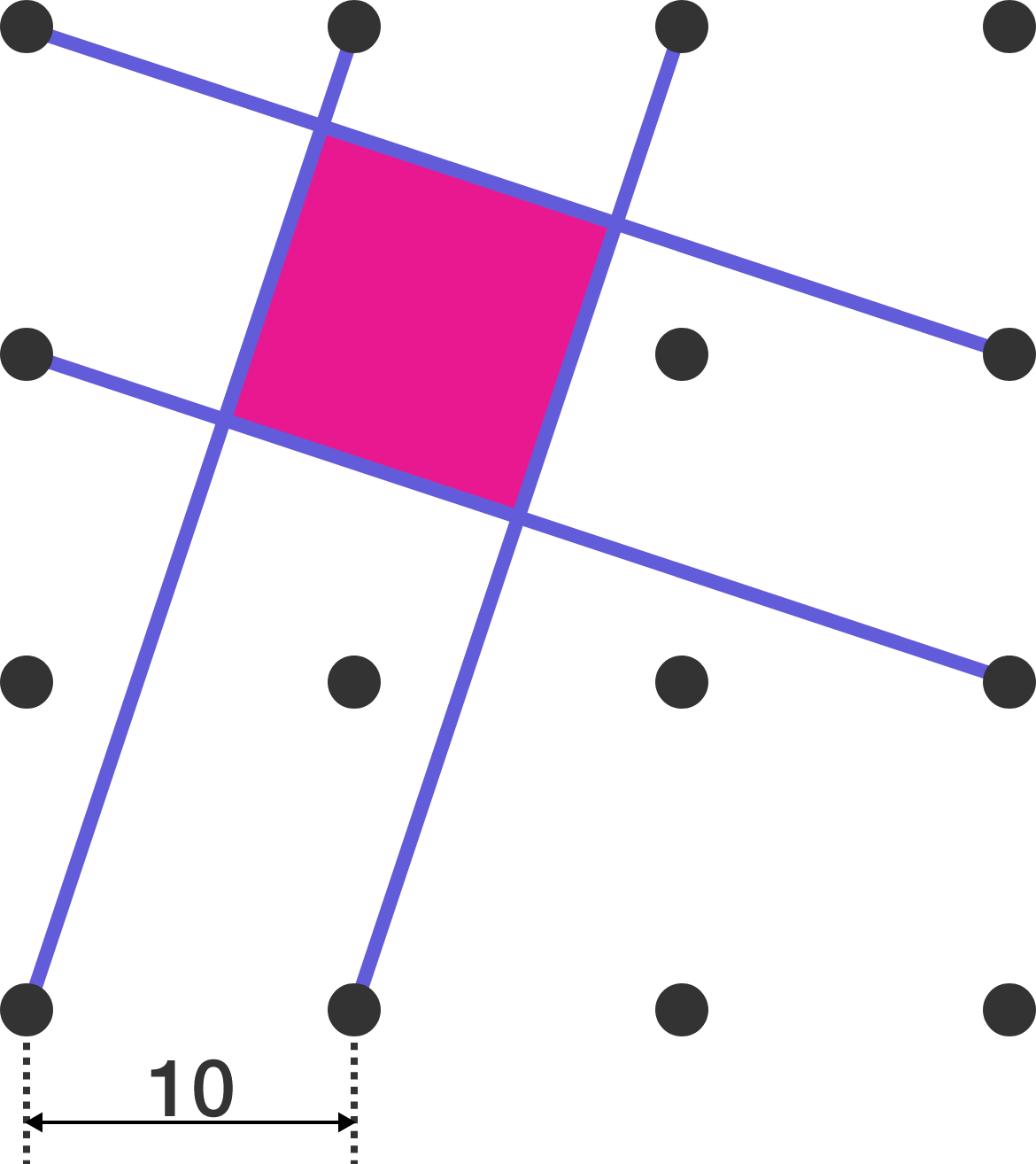# Problems of the Week

Contribute a problem

# 2017-07-24 Basic

If ${\color{#3D99F6}{a}},$ ${\color{#20A900}{b}},$ and ${\color{#D61F06}{c}}$ are integers, is it possible that ${\color{#3D99F6}{a}}+{\color{#20A900}{b}},$ ${\color{#3D99F6}{a}}+{\color{#D61F06}{c}},$ and ${\color{#20A900}{b}}+{\color{#D61F06}{c}}$ are all odd numbers?


Note: It is possible for all three sums to be even!

What number goes into the blank below?

${\color{#3D99F6}1} + {\color{#D61F06}\dfrac12} + {\color{#3D99F6}\dfrac14} + {\color{#D61F06}\dfrac18} + {\color{#3D99F6}\dfrac1{16}} + \cdots =\left({\color{#3D99F6}1} - {\color{#D61F06}\dfrac12} + {\color{#3D99F6}\dfrac14} - {\color{#D61F06}\dfrac18} + {\color{#3D99F6}\dfrac1{16}} - \cdots \right) \times \text{\_\_\_\_\_\_\_}$A Ferris wheel rotates with constant angular speed, which causes the people inside the cabins to feel forces on their bodies. To remain seated in the same position, they hold tightly onto the cabin's seat, floor, and grab bar, using all parts of their body.

At what point in the revolution of the Ferris wheel do they need to exert the greatest net force on the cabin?

What is $x,$ in meters?The cue ball starts 0.25 m below the top wall and 0.25 m right of the left wall. Assume that the ball and pocket are of point size and have no width, and that the angle of incidence equals the angle of reflection when the ball hits the wall.

Note: Iranian mathematician and Fields medalist Maryam Mirzakhani passed away on July 14, 2017 after a long battle with cancer. This is a tribute to her outstanding work in geometry and billiards dynamics.

On the regular grid below, four line segments form the pink square. What is its area?×

Problem Loading...

Note Loading...

Set Loading...